# Activity 2 Activity 1 Index Student Activity 1

• Slides: 9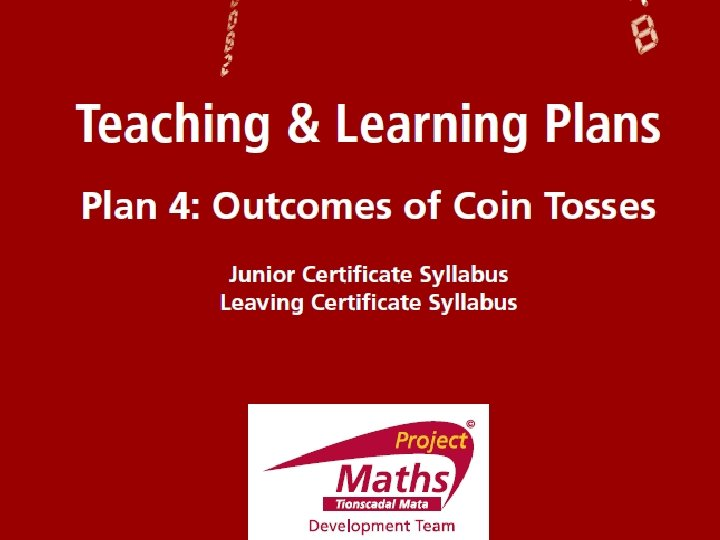Activity 2 Activity 1 Index Student Activity 1: Tossing a coin Student Activity 2: Tossing two coins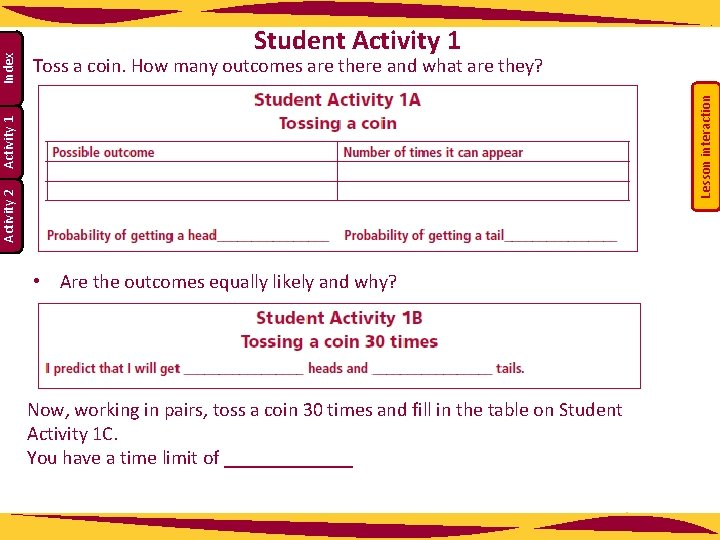Lesson interaction Toss a coin. How many outcomes are there and what are they? Activity 2 Activity 1 Index Student Activity 1 • Are the outcomes equally likely and why? Now, working in pairs, toss a coin 30 times and fill in the table on Student Activity 1 C. You have a time limit of _______Lesson interaction Index Activity 1 Activity 2 Now, working in pairs, toss a coin 30 times and fill in the table on Student Activity 1 C. You have a time limit of _____Lesson interaction Index Activity 1 Activity 2 • Why are the results now closer to the predicted values? • If I get four heads in a row does that increase the chances of getting a tail next time? • Can you think of any other real life situations which have only 2 possible outcomes?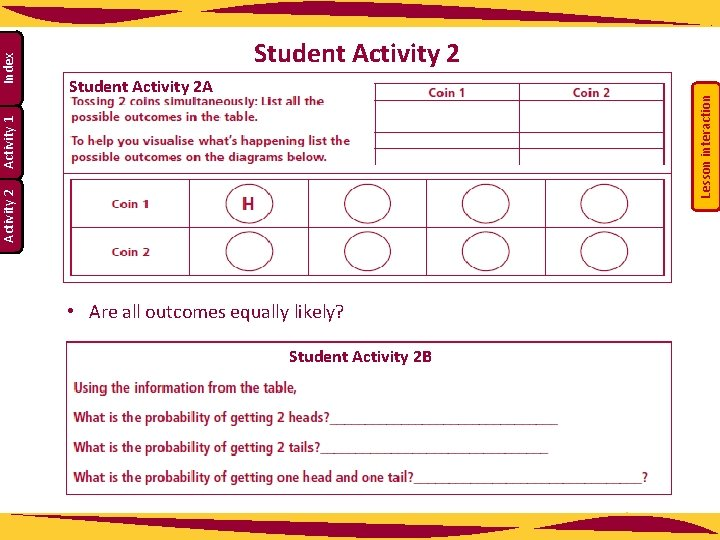Lesson interaction Student Activity 2 A Activity 2 Activity 1 Index Student Activity 2 • Are all outcomes equally likely? Student Activity 2 B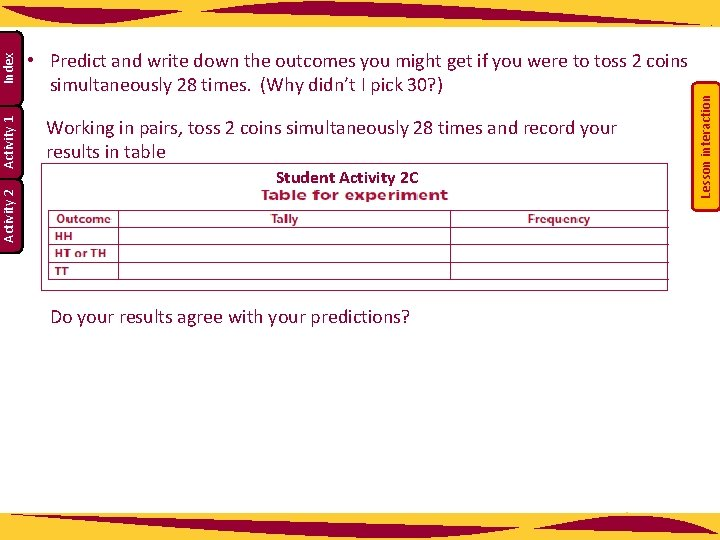Student Activity 2 C Do your results agree with your predictions? Lesson interaction Working in pairs, toss 2 coins simultaneously 28 times and record your results in table Index Activity 2 Activity 1 • Predict and write down the outcomes you might get if you were to toss 2 coins simultaneously 28 times. (Why didn’t I pick 30? )• Are these results in agreement with your predictions? Lesson interaction Index Activity 2 Activity 1 Student Activity 2 D • The table only gives one option for heads and tails combination, while the origina table showed HT and TH. Will this make a difference to the results? • Why? • How does this fit in with what you learned in the fundamental principle of counting?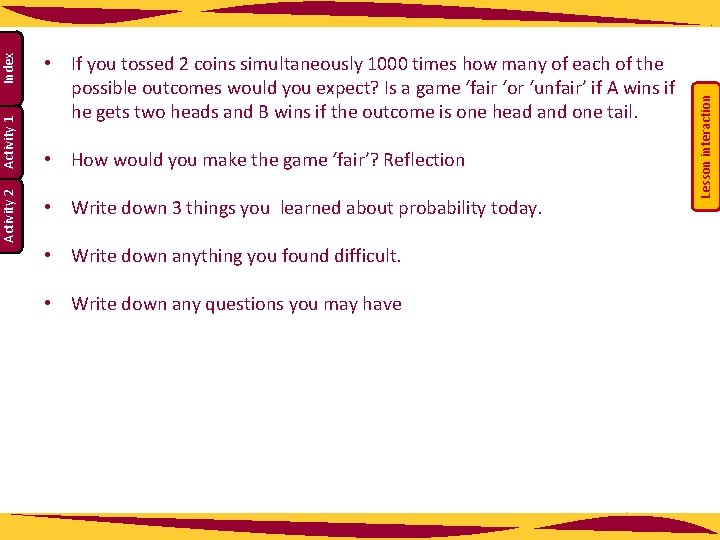• How would you make the game ‘fair’? Reflection • Write down 3 things you learned about probability today. • Write down anything you found difficult. • Write down any questions you may have Lesson interaction Index Activity 1 Activity 2 • If you tossed 2 coins simultaneously 1000 times how many of each of the possible outcomes would you expect? Is a game ‘fair ‘or ‘unfair’ if A wins if he gets two heads and B wins if the outcome is one head and one tail.